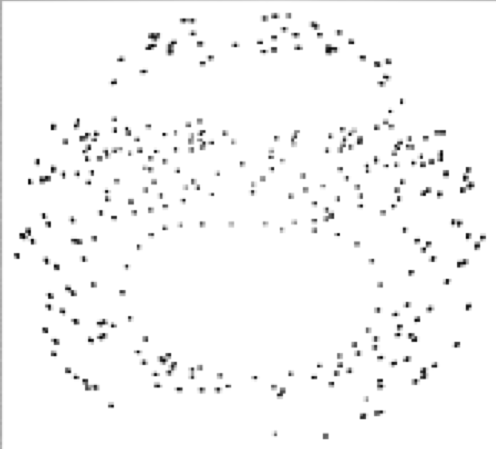# The SonEnvir project portal

##### Personal tools
You are here: Home data Chaotic double pendulum (Physics)

# Double Pendulum - sonification details

### Introduction

The tutorial for this project is taken from the book: An Introduction to Computer Simulation Methods, 2nd Edition, Harvey Gould, Jan Tobochnik, Addison Wesley, 1996. It was elaborated in a course on computational physics by Prof. Chr. Gattringer.

A pendulum (consisting of a weight of mass m and a massless cord of length l) is hanged on a solid ceiling; on this first a second, identical pendulum is fixed. The system has two degrees of freedom: it may only move in a plane. Energy is conserved (there is no friction), thus it is a hamiltonian system, which may be described by its canonical momenta, p_1 and p_2 respectively, and its canonical coordinates (the angles),q_1 and q_2. Depending on the initial conditions, the system behaves regularly or shows chaotic evolution.

This physical system is numerically modeled using the forth-order Runge-Kutta Method. This is an iterative method for solving differential equations numerically, the error of the solution being of the order of the step size to the power of 5.

### Classical Analysis vs. sonficationIn a visualization, usually a Poincare-plot is done. Two parameters of the system are chosen, and the values of the others are fixed in phase space: e.g. in this plot, points were plotted whenever q_2 = 0 and p_2 > 0. A regular behavior would show as closed loops in such a plot. The plot here shows chaotic behavior; some regions are never reached by the system, and this may be interpreted. In a Poincare plot, there is no time axis.

In a sonification, one has the possibility to keep the physical time and still display all of the system's parameters: this was done by mapping the angles to frequencies (modulating two sine waves) and the coordinates to the amplitude of the two channels. Regular behavior results in a periodic signal and may easily be distinguished from a quasi-periodic and chaotic signal. Also another sonification using rhythm was done.

### References

For a detailed description please refer to Project description (pdf) .